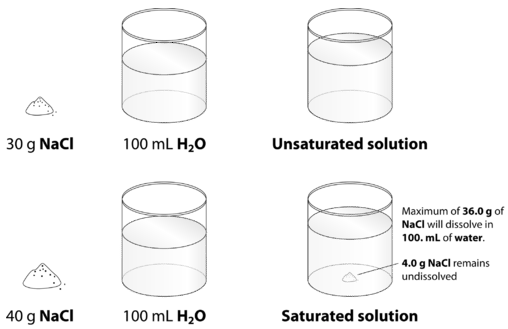# 16.3: Saturated and Unsaturated Solutions

$$\newcommand{\vecs}{\overset { \rightharpoonup} {\mathbf{#1}} }$$ $$\newcommand{\vecd}{\overset{-\!-\!\rightharpoonup}{\vphantom{a}\smash {#1}}}$$$$\newcommand{\id}{\mathrm{id}}$$ $$\newcommand{\Span}{\mathrm{span}}$$ $$\newcommand{\kernel}{\mathrm{null}\,}$$ $$\newcommand{\range}{\mathrm{range}\,}$$ $$\newcommand{\RealPart}{\mathrm{Re}}$$ $$\newcommand{\ImaginaryPart}{\mathrm{Im}}$$ $$\newcommand{\Argument}{\mathrm{Arg}}$$ $$\newcommand{\norm}{\| #1 \|}$$ $$\newcommand{\inner}{\langle #1, #2 \rangle}$$ $$\newcommand{\Span}{\mathrm{span}}$$ $$\newcommand{\id}{\mathrm{id}}$$ $$\newcommand{\Span}{\mathrm{span}}$$ $$\newcommand{\kernel}{\mathrm{null}\,}$$ $$\newcommand{\range}{\mathrm{range}\,}$$ $$\newcommand{\RealPart}{\mathrm{Re}}$$ $$\newcommand{\ImaginaryPart}{\mathrm{Im}}$$ $$\newcommand{\Argument}{\mathrm{Arg}}$$ $$\newcommand{\norm}{\| #1 \|}$$ $$\newcommand{\inner}{\langle #1, #2 \rangle}$$ $$\newcommand{\Span}{\mathrm{span}}$$$$\newcommand{\AA}{\unicode[.8,0]{x212B}}$$

When compounds are synthesized, contaminating materials are often mixed in with them. The process of recrystallization can be used to remove these impurities. The crystals are dissolved in a hot solvent, forming a solution. When the solvent is cooled, the compound is no longer as soluble and will precipitate out of solution, leaving contaminating materials still dissolved.

## Saturated and Unsaturated Solutions

Table salt $$\left( \ce{NaCl} \right)$$ readily dissolves in water. Suppose that you have a beaker of water to which you add some salt, stirring until it dissolves. You add more and that dissolves. You keep adding more and more salt, eventually reaching a point at which no more of the salt will dissolve—no matter how long or how vigorously you stir it. Why? On the molecular level, we know that action of the water causes the individual ions to break apart from the salt crystal and enter the solution, where they remain hydrated by water molecules. What also happens is that some of the dissolved ions collide back again with the crystal and remain there. Recrystallization is the process of dissolved solute returning to the solid state. At some point, the rate at which the solid salt is dissolving becomes equal to the rate at which the dissolved solute is recrystallizing. When that point is reached, the total amount of dissolved salt remains unchanged. Solution equilibrium is the physical state described by the opposing processes of dissolution and recrystallization occurring at the same rate. The solution equilibrium for the dissolution of sodium chloride can be represented by one of two equations:

$\ce{NaCl} \left( s \right) \rightleftharpoons \ce{NaCl} \left( aq \right)\nonumber$

While the above equation shows the change of state back and forth between solid and aqueous solution, the preferred equation also shows the dissociation that occurs as an ionic solid dissolves:

$\ce{NaCl} \left( s \right) \rightleftharpoons \ce{Na^+} \left( aq \right) + \ce{Cl^-} \left( aq \right)\nonumber$

When the solution equilibrium point is reached and no more solute will dissolve, the solution is said to be saturated. A saturated solution is a solution that contains the maximum amount of solute that is capable of dissolving. At $$20^\text{o} \text{C}$$, the maximum amount of $$\ce{NaCl}$$ that will dissolve in \100. \: \text{g}\) of water is $$36.0 \: \text{g}$$. If any more $$\ce{NaCl}$$ is added past this point, it will not dissolve, because the solution is saturated. What if more water is added to the solution instead? In that case, more $$\ce{NaCl}$$ would be capable of dissolving in the additional solvent. An unsaturated solution is a solution that contains less than the maximum amount of solute that is capable of being dissolved. The figure below illustrates the above process and shows the distinction between unsaturated and saturated.Figure $$\PageIndex{1}$$: When $$30.0 \: \text{g}$$ of $$\ce{NaCl}$$ is added to $$100 \: \text{mL}$$, it all dissolves, forming an unsaturated solution. When $$40.0 \: \text{g}$$ is added, $$36.0 \: \text{g}$$ dissolves and $$4.0 \: \text{g}$$ remains undissolved, forming a saturated solution.

How can you tell if a solution is saturated or unsaturated? If more solute is added and it does not dissolve, then the original solution was saturated. If the added solute dissolves, then the original solution was unsaturated. A solution that has been allowed to reach equilibrium, but which has extra undissolved solute at the bottom of the container, must be saturated.

## Summary

• Recrystallization is the process of dissolved solute returning to the solid state.
• A saturated solution is a solution that contains the maximum amount of solute that is capable of dissolving.
• An unsaturated solution is a solution that contains less than the maximum amount of solute that is capable of being dissolved.
• Solution equilibrium exists when the rate of dissolution equals the rate of recrystallization.

This page titled 16.3: Saturated and Unsaturated Solutions is shared under a CK-12 license and was authored, remixed, and/or curated by CK-12 Foundation via source content that was edited to the style and standards of the LibreTexts platform; a detailed edit history is available upon request.